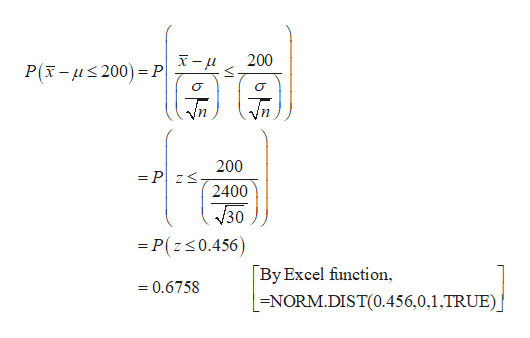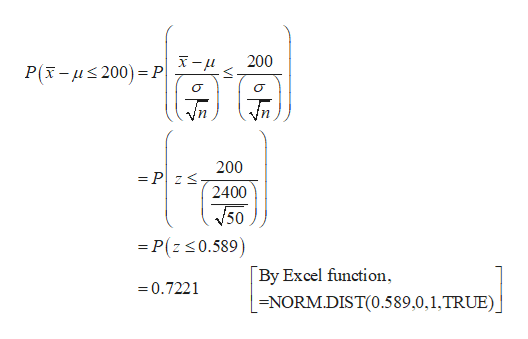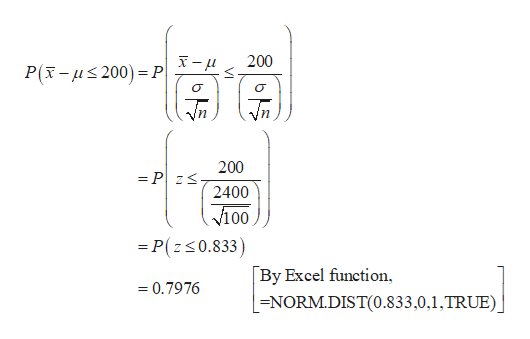# The Wall Street Journal reports that 33% of taxpayers with adjusted gross incomes between \$30,000 and \$60,000 itemized deductions on their federal income tax return. The mean amount of deductions for this population of taxpayers was \$16,642. Assume the standard deviation is σ = \$2,400.(a)What is the probability that a sample of taxpayers from this income group who have itemized deductions will show a sample mean within \$200 of the population mean for each of the following sample sizes: 30, 50, 100, and 500? (Round your answers to four decimal places.)sample size n = 30sample size n = 50sample size n = 100sample size n = 500(b)What is the advantage of a larger sample size when attempting to estimate the population mean?A larger sample lowers the population standard deviation.A larger sample increases the probability that the sample mean will be a specified distance away from the population mean.    A larger sample has a standard error that is closer to the population standard deviation.A larger sample increases the probability that the sample mean will be within a specified distance of the population mean.

Question
39 views
The Wall Street Journal reports that 33% of taxpayers with adjusted gross incomes between \$30,000 and \$60,000 itemized deductions on their federal income tax return. The mean amount of deductions for this population of taxpayers was \$16,642. Assume the standard deviation is
σ = \$2,400.
(a)
What is the probability that a sample of taxpayers from this income group who have itemized deductions will show a sample mean within \$200 of the population mean for each of the following sample sizes: 30, 50, 100, and 500? (Round your answers to four decimal places.)
sample size n = 30sample size n = 50sample size n = 100sample size n = 500
(b)
What is the advantage of a larger sample size when attempting to estimate the population mean?
A larger sample lowers the population standard deviation.A larger sample increases the probability that the sample mean will be a specified distance away from the population mean.    A larger sample has a standard error that is closer to the population standard deviation.A larger sample increases the probability that the sample mean will be within a specified distance of the population mean.
check_circle

Step 1

(a)

The probability that a sample of taxpayers from this income group who have itemized deductions will show a sample mean within \$200 of the population mean is calculated below:

When sample size n=30:

From the given information, the population mean is 16,642 and standard deviation is 2,400.help_outlineImage Transcriptionclose200 P(x- us 200) =P 200 = P zs. 2400 30 = P(zs0.456) [By Excel function, =NORM.DIST(0.456,0,1,TRUE)] = 0.6758 VI |아두 fullscreen
Step 2

When sample size n=50:help_outlineImage Transcriptionclose200 P(x- us 200) =P 200 = P z<. 2400 V50 = P(z <0.589) [By Excel function, = 0.7221 =NORM.DIST(0.589,0,1,TRUE). VI fullscreen
Step 3

When sample size n=100:

...help_outlineImage Transcriptionclose200 P(x- us 200) =P 200 = P z<. 2400 V100 = P(z<0.833) By Excel function, = 0.7976 =NORM.DIST(0.833,0,1,TRUE) VI |아들 fullscreen

### Want to see the full answer?

See Solution

#### Want to see this answer and more?

Solutions are written by subject experts who are available 24/7. Questions are typically answered within 1 hour.*

See Solution
*Response times may vary by subject and question.
Tagged in

### Other